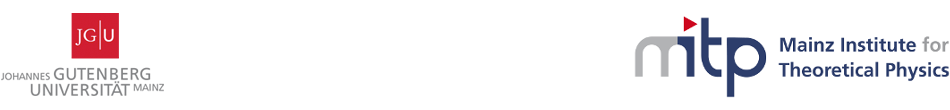Geometry, Gravity and Supersymmetry

24-28 April 2017
Mainz Institute for Theoretical Physics, Johannes Gutenberg University
Europe/Berlin timezone

Duality and the Generalised Geometry of (2,1) Sigma Models in Superspace

25 Apr 2017, 16:30
30m
02.430 (Mainz Institute for Theoretical Physics, Johannes Gutenberg University)

02.430

Mainz Institute for Theoretical Physics, Johannes Gutenberg University

Staudingerweg 9 / 2nd floor, 55128 Mainz

Speaker

Dr Mohab Abou Zeid (Georg-August-Universität Göttingen)

Description

The geometry, isometry symmetries and superspace formulation of two-dimensional non-linear sigma models with manifest (2,1) supersymmetry is revisited. Following Rocek and Verlinde, we then dualise the models by gauging the isometries using suitable (2,1) gauge multiplets and introducing Lagrange multiplier superfields that constrain the field strengths of the gauge multiplets to vanish. Integrating out the Lagrange multipliers leads to the original action, whereas integrating out the vector multiplets yields the dual action. The geometry of the dual model is defined implcitly in terms of the appropriate vector superfield potentials. Superspace reductions and the relation to the usual Buscher rules are investigated.

Primary author

Dr Mohab Abou Zeid (Georg-August-Universität Göttingen)

 Photo